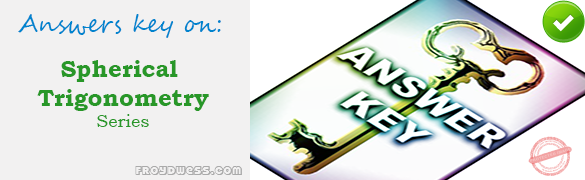# MCQs in Spherical Trigonometry Part I – Answers

(Last Updated On: December 8, 2017)Below are the answers key for the Multiple Choice Questions in Spherical Trigonometry – MCQs Part 1.

1. B. 8 A.M.

Review: Solution for Number 1

2. A. 1 hour and 12 minutes

Review: Solution for Number 2

3. B. 4 minutes

Review: Solution for Number 3

4. B. 74.33°

Review: Solution for Number 4

5. C. 168º 31’

Review: Solution for Number 5

6. B. 68º

Review: Solution for Number 6

7. C. 86º 15’

Review: Solution for Number 7

8. D. 41º 45’

Review: Solution for Number 8

9. C. 97º 09’

Review: Solution for Number 9

10. D. 119º

Review: Solution for Number 10

11. A. 33º 33’

Review: Solution for Number 11

12. B. 90º

Review: Solution for Number 12

13. B. 16531 sq. m.

Review: Solution for Number 13

14. C. 25 km

Review: Solution for Number 14

15. C. 16.4

Review: Solution for Number 15

16. D. 15.27 sq. m.

17. C. 74.33°

18. C. 4 p.m.

19. A. 2.87 hours

20. D. 6046.2 nautical miles

### Online Questions and Answers in Spherical Trigonometry Series

Following is the list of practice exam test questions in this brand new series:

Plane Trigonometry MCQs
PART 1: MCQs from Number 1 – 50                        Answer key: PART I
MCQs in Spherical Trigonometry Part I – Answers
5 (100%) 1 vote